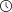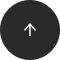- 8 minutes read

# High-Performance Statistical Queries: Dependencies Between Discrete Variables

In my previous article, we looked at how you can calculate linear dependencies between two continuous variables with covariance and correlation. Both methods use the means of the two variables in their calculations. However, mean values and other population moments make no sense for categorical (nominal) variables.

For instance, if you denote "Clerical" as 1 and "Professional" as 2 for an occupation variable, what does the average of 1.5 signify? You have to find another test for dependencies—a test that does not rely on numeric values. You can use contingency tables and the chi-squared test. Let's take a closer look at these two methods.

### Contingency Tables

Contingency tables are used to examine the relationship between subject scores for two qualitative or categorical variables. They show the actual and expected distributions of cases in a cross-tabulated (pivoted) format for the two variables.

Let's start with a simple pivot table. Table 1 below shows the number of cases, or the actual distribution of cases, over the Occupation column (on rows) and the Marital Status column (on columns).

TABLE 1 A pivot table example

Occupation \ Marital Status Married Single Total
Clerical 4745 4388 9133
Professional 5266 4085 9351
Total 10011 8473 18484

If the two variables are independent, then the actual distribution in every single cell should be approximately equal to the expected distribution for that cell. The expected distribution is obtained by calculating the marginal probability. For example, the marginal probability of the value "Married" is 10111 / 18484 = 0.5416. This tells us that more than 54% of the people in our sample are married. If the two variables were independent, then you would expect to have approximately 54% of married people among clericals and 54% among professionals. You can of course determine if a dependency exists between two discrete variables by just viewing their contingency table. However, a solid numerical measure is often preferred.

Table 2 below shows the actual (observed) and expected distributions of cases over the Occupation column (on rows) and the Marital Status column (on columns):

TABLE 2 A contingency table example

Occupation \ Marital Status Married Single Total Actual
Expected
Clerical           Actual
Expected
4745
4946
4388
4187
9133
9133
Professional    Actual
Expected
5266
5065
4085
4286
9351
9351
Total                Actual
Expected
10011
10111
8473
8473
18484
18484

### Chi-Squared Test

If the columns are not contingent on the rows, then the row and column frequencies are independent. The test of whether columns are contingent on rows is called the chi-squared test of independence. The null hypothesis for this test is that there is no relationship between row and column frequencies—in other words, that the variables are independent. Therefore, there should be no difference between the observed (O) and expected (E) frequencies.

The chi-squared test simply calculates the sum of the squares of differences between observed and expected frequencies divided by expected frequencies. This formula is also referred to as the Pearson chi-squared formula.There exist premade tables that list the critical points for chi-squared distributions. If your calculated chi-squared value is greater than a critical value in such a table for the defined degrees of freedom and for a specific confidence level, you can reject the null hypothesis with that confidence. For a table with R rows and C columns, the degree of freedom is calculated as the following product:Table 3 lists the critical values of the chi-squared distribution. Greater differences between expected and actual data produce larger chi-squared values. A greater chi-squared value suggests that there is a higher probability that there really is a significant difference between expected and observed frequencies. The Probability row at the bottom of the table indicates the maximum probability that the null hypothesis holds when the chi-squared value you calculate is greater than or equal to the value listed in the table for your particular degrees of freedom.

TABLE 3 Chi-squared Critical Points

DF Chi-squared Value
1 0.004 0.02 0.06 0.15 0.46 1.07 1.64 2.71 3.84 6.64 10.83
2 0.10 0.21 0.45 0.71 1.39 2.41 3.22 4.60 5.99 9.21 13.82
3 0.35 0.58 1.01 1.42 2.37 3.66 4.64 6.25 7.82 11.34 16.27
4 0.71 1.06 1.65 2.20 3.36 4.88 5.99 7.78 9.49 13.28 18.47
5 1.14 1.61 2.34 3.00 4.35 6.06 7.29 9.24 11.07 15.09 20.52
6 1.63 2.20 3.07 3.83 5.35 7.23 8.56 10.64 12.59 16.81 22.46
7 2.17 2.83 3.82 4.67 6.35 8.38 9.80 12.02 14.07 18.48 24.32
8 2.73 3.49 4.59 5.53 7.34 9.52 11.03 13.56 15.51 20.09 26.12
9 3.32 4.17 5.38 6.39 8.34 10.66 12.24 14.68 16.92 21.67 27.88
10 3.94 4.86 6.18 7.27 9.34 11.78 13.44 15.99 18.31 23.21 29.59
Probability 0.95 0.90 0.80 0.70 0.50 0.30 0.20 0.10 0.05 0.01 0.001
Not significant       Significant

For example, suppose you calculate a chi-squared value for two discrete variables. Let's say the chi-squared value you get is 16, and the degrees of freedom are 7. Scan the seventh row of Table 2 above for the first chi-squared value that is greater than the one you calculated. In this case, that value is 14.07. Do the same for the first chi-squared value that is greater than yours, which happens to be 18.48 here.

Now, check the corresponding probabilities for these two values—0.05 and 0.01, respectively. This means that there is a less than 5% probability that the two variables are independent and more than 1% probably that they are independent. This is a significant result, and you can say with more than 95% confidence that the two variables are dependent.

### Chi-Squared Calculation: a Naive Approach

Calculating a chi-squared value is not that simple with T-SQL. The difficulty is not in obtaining the observed frequencies but rather calculating the expected frequencies. You can calculate expected frequencies from marginal frequencies or from the totals over rows and columns. Let's do this for the `NumberCarsOwned` and `MaritalStatus` variables from the `dbo.vTargetMail` view of the `AdventureWorksDW2014` demo database.

The query below does this step by step. The first common table expression (CTE) calculates the observed frequencies for the combination of both variables' states using grouping by both variables (`NumberCarsOwned` and `MaritalStatus`). The second CTE calculates the observed frequencies for the first variable, `NumberCarsOwned`, while the third CTE calculates the observed frequencies for the second variable, `MaritalStatus`. Moving on, the fourth CTE calculates the observed total frequencies (total number of cases). Finally, the last CTE joins together the observed and expected frequencies so the outer query can calculate the chi-squared value and the degrees of freedom.

```WITH
ObservedCombination_CTE AS
(
SELECT NumberCarsOwned, MaritalStatus, COUNT(*) AS Observed
FROM dbo.vTargetMail
GROUP BY NumberCarsOwned, MaritalStatus
),
ObservedFirst_CTE AS
(
SELECT NumberCarsOwned, NULL AS MaritalStatus, COUNT(*) AS Observed
FROM dbo.vTargetMail
GROUP BY NumberCarsOwned
),
ObservedSecond_CTE AS
(
SELECT NULL AS NumberCarsOwned, MaritalStatus, COUNT(*) AS Observed
FROM dbo.vTargetMail
GROUP BY MaritalStatus
),
ObservedTotal_CTE AS
(
SELECT NULL AS NumberCarsOwned, NULL AS MaritalStatus, COUNT(*) AS Observed
FROM dbo.vTargetMail
),
ExpectedCombination_CTE AS
(
SELECT F.NumberCarsOwned, S.MaritalStatus,
CAST(ROUND(1.0 * F.Observed * S.Observed / T.Observed, 0) AS INT) AS Expected
FROM ObservedFirst_CTE AS F
CROSS JOIN ObservedSecond_CTE AS S
CROSS JOIN ObservedTotal_CTE AS T
),
ObservedExpected_CTE AS
(
SELECT O.NumberCarsOwned, O.MaritalStatus, O.Observed, E.Expected
FROM ObservedCombination_CTE AS O
INNER JOIN ExpectedCombination_CTE AS E
ON O.NumberCarsOwned = E.NumberCarsOwned
AND O.MaritalStatus = E.MaritalStatus
)
SELECT SUM(SQUARE(1.0 * Observed - Expected) / Expected) AS ChiSquared,
(COUNT(DISTINCT NumberCarsOwned) - 1) *
(COUNT(DISTINCT MaritalStatus) - 1) AS DegreesOfFreedom
FROM ObservedExpected_CTE;
```

I won't show the result of the above query, as it's the same as the next one in this article. The next query performs much better—we'll see why in a moment.

### Chi-Squared Calculation: an Efficient Approach

The query we developed above is fairly inefficient, as it scans our data many times. I only showed that query to help you more easily understand the process of calculating expected frequencies with T-SQL. Fortunately, from SQL Server 2012 onward, you can use window aggregate functions to make this calculation much simpler.

The following query uses only two common table expressions: the first one just calculates the observed frequencies for the combination of both variables' states, and the second one uses the window aggregate functions to calculate the marginal and total frequencies and the expected frequencies for the combination of both variables' states. The outer query calculates the chi-squared and the degrees of freedom.

```WITH
ObservedCombination_CTE AS
(
SELECT NumberCarsOwned AS OnRows,
MaritalStatus AS OnCols,
COUNT(*) AS ObservedCombination
FROM dbo.vTargetMail
GROUP BY NumberCarsOwned, MaritalStatus
),
ExpectedCombination_CTE AS
(
SELECT OnRows, OnCols, ObservedCombination
,SUM(ObservedCombination) OVER (PARTITION BY OnRows) AS ObservedOnRows
,SUM(ObservedCombination) OVER (PARTITION BY OnCols) AS ObservedOnCols
,SUM(ObservedCombination) OVER () AS ObservedTotal
,CAST(ROUND(SUM(1.0 * ObservedCombination) OVER (PARTITION BY OnRows)
* SUM(1.0 * ObservedCombination) OVER (PARTITION BY OnCols)
/ SUM(1.0 * ObservedCombination) OVER (), 0) AS INT) AS ExpectedCombination
FROM ObservedCombination_CTE
)
SELECT SUM(SQUARE(ObservedCombination - ExpectedCombination)
/ ExpectedCombination) AS ChiSquared,
(COUNT(DISTINCT OnRows) - 1) * (COUNT(DISTINCT OnCols) - 1) AS DegreesOfFreedom
FROM ExpectedCombination_CTE;
```

The result of this query is

```ChiSquared             DegreesOfFreedom
---------------------- ----------------
304.301222593159       4
```

Now, you can read the chi-squared critical points table. For four degrees of freedom, locate the highest value in Table 3, which you can find in the last column of the fourth row: 18.47. The probability for this value is 0.001. Note that our measured chi-squared value is 304.30, which is much higher than the 18.47. You can therefore say with more than 99.9% confidence that the `NumberCarsOwned` and `MaritalStatus` variables are dependent. Naturally, married people tend to have bigger families with more cars.

### Conclusion

You have seen a practical usage of the window aggregate functions and some common table expressions. If you'd like to learn more about these two topics, I highly recommend you to take Window Functions and Recursive Queries courses.

So far, we've covered how to measure the strength of the association between two continuous and two discrete variables. In a future article, we'll take a look at measuring the level of association between one continuous and one discrete variable. Stay tuned!